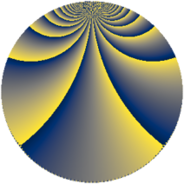# Properties

 Label 1216.4.bsLevel $1216$ Weight $4$ Character orbit 1216.bs Rep. character $\chi_{1216}(15,\cdot)$ Character field $\Q(\zeta_{36})$ Dimension $1416$ Sturm bound $640$

# Related objects

## Defining parameters

 Level: $$N$$ $$=$$ $$1216 = 2^{6} \cdot 19$$ Weight: $$k$$ $$=$$ $$4$$ Character orbit: $$[\chi]$$ $$=$$ 1216.bs (of order $$36$$ and degree $$12$$) Character conductor: $$\operatorname{cond}(\chi)$$ $$=$$ $$304$$ Character field: $$\Q(\zeta_{36})$$ Sturm bound: $$640$$

## Dimensions

The following table gives the dimensions of various subspaces of $$M_{4}(1216, [\chi])$$.

Total New Old
Modular forms 5856 1464 4392
Cusp forms 5664 1416 4248
Eisenstein series 192 48 144

## Trace form

 $$1416q + 12q^{3} - 12q^{5} + 12q^{7} + O(q^{10})$$ $$1416q + 12q^{3} - 12q^{5} + 12q^{7} + 6q^{11} - 12q^{13} - 24q^{17} + 12q^{19} + 150q^{21} + 24q^{23} + 18q^{27} - 12q^{29} - 24q^{33} - 738q^{35} + 48q^{39} + 12q^{43} - 6q^{45} - 31176q^{49} - 2220q^{51} - 12q^{53} + 24q^{55} + 12q^{59} - 12q^{61} - 36q^{65} + 12q^{67} - 4770q^{69} + 24q^{71} + 4092q^{77} - 24q^{81} + 6q^{83} - 1452q^{85} + 12q^{87} - 2046q^{91} + 150q^{93} - 24q^{97} + 4386q^{99} + O(q^{100})$$

## Decomposition of $$S_{4}^{\mathrm{new}}(1216, [\chi])$$ into newform subspaces

The newforms in this space have not yet been added to the LMFDB.

## Decomposition of $$S_{4}^{\mathrm{old}}(1216, [\chi])$$ into lower level spaces

$$S_{4}^{\mathrm{old}}(1216, [\chi]) \cong$$ $$S_{4}^{\mathrm{new}}(304, [\chi])$$$$^{\oplus 3}$$